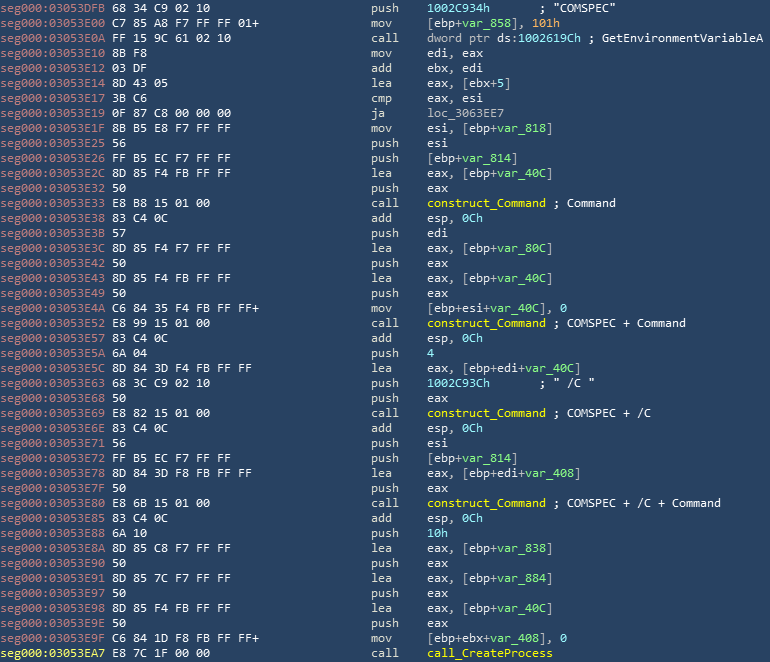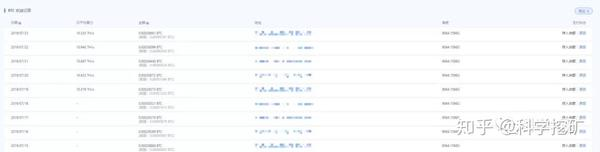# 区块链的作业是比特币挖矿程序python实现，我做完了

6个月前 (12-01) 66次浏览```#Author:Mr.Pan_学狂
#Finish_time:2020/11/22/22:34
import hashlib
import time
def find_gold():#参数D是难度系数
x = 0#算力初始值
Diffcult_number = str(input('请输入难度系数：'))
print('开始挖矿!!')
while True:
x += 1
string = 'hello'
start_string = str(x)
combine_string = start_string + string#字符串拼接
h = hashlib.sha256()#采用sha256加密
h.update(combine_string.encode('utf-8'))#utf-8编码后哈希加密
gold_hash = h.hexdigest()
#print(gold_hash)
startTime = time.perf_counter()
if gold_hash[0:len(Diffcult_number)] == Diffcult_number:#对比哈希值的x位与难度系数是否相同，x为难度系数
print('算力:{}'.format(x), '用时:{}'.format(startTime))
print('哈希值:%s' % gold_hash)#占位符
print('挖矿成功！！')
break
elif gold_hash[0:len(Diffcult_number)] != Diffcult_number:#哈希值前x位与难度系数不相同
print('算力:{}'.format(x),'用时:{}'.format(startTime))
print('哈希值:%s' % gold_hash)
print('没有挖到！！')
print()
continue
if __name__ == '__main__':#主函数,调用函数，程序出口。
find_gold()```